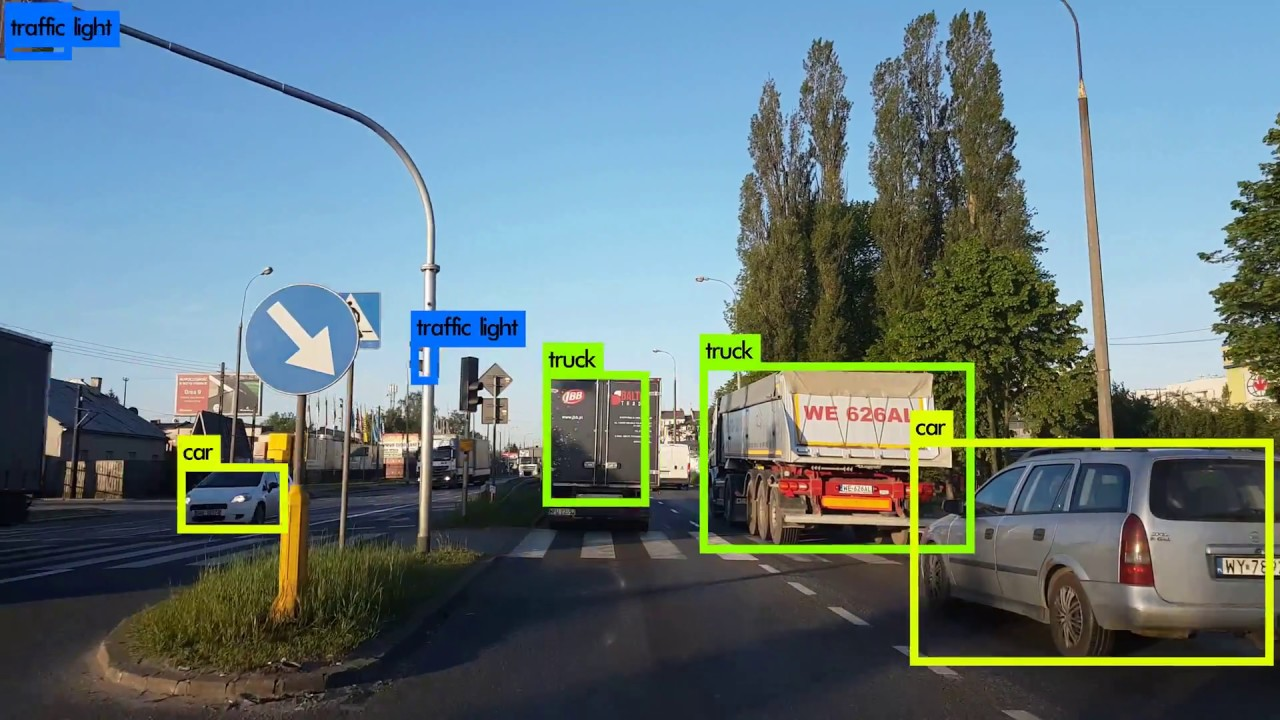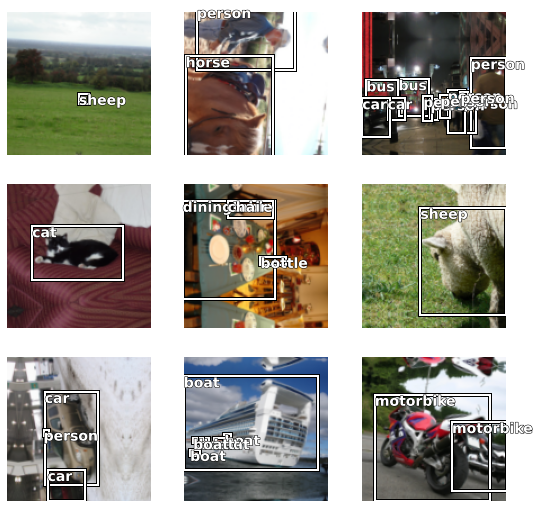Lesson Video:

This article is also a Jupyter Notebook available to be run from the top down. There will be code snippets that you can then run in any environment.

Below are the versions of fastai, fastcore, and wwf currently running at the time of writing this:

• fastai: 2.1.10
• fastcore: 1.3.13
• wwf: 0.0.8

Object Detection

Finding the localized area in which an object presides from two points, the bottom left and top rightfrom fastai.vision.all import *
path = untar_data(URLs.PASCAL_2007)

Now how do we get our labels? fastai has a get_annotations function that we can use to grab the image and their bounding box. The one-line documentation states: "Open a COCO style json in fname and returns the list of filenames (with mabye prefix) and labelled bounding boxes."

path.ls()
(#8) [Path('/root/.fastai/data/pascal_2007/valid.json'),Path('/root/.fastai/data/pascal_2007/test.json'),Path('/root/.fastai/data/pascal_2007/test.csv'),Path('/root/.fastai/data/pascal_2007/train'),Path('/root/.fastai/data/pascal_2007/test'),Path('/root/.fastai/data/pascal_2007/train.csv'),Path('/root/.fastai/data/pascal_2007/segmentation'),Path('/root/.fastai/data/pascal_2007/train.json')]

We'll want to read out of the train.json

imgs, lbl_bbox = get_annotations(path/'train.json')
imgs
'000012.jpg'
lbl_bbox
([[155, 96, 351, 270]], ['car'])

Next, we want to be able to quickly look up a corresponding image to it's label. We'll use a dictionary

img2bbox = dict(zip(imgs, lbl_bbox))

Let's check the first item

first = {k: img2bbox[k] for k in list(img2bbox)[:1]}; first
{'000012.jpg': ([[155, 96, 351, 270]], ['car'])}

Great! Now let's build our DataBlock. We'll have two outputs, the bounding box itself and a label, with one input.

getters = [lambda o: path/'train'/o, lambda o: img2bbox[o], lambda o: img2bbox[o]]

For our transforms, we'll use some of the ones we defined earlier

batch_tfms = [Rotate(), Flip(), Dihedral(), Normalize.from_stats(*imagenet_stats)]

Why do we need a custom get_images? Because we want our images that came back to us, not the entire folder

def get_train_imgs(noop):  return imgs

We'll now make our DataBlock. We want to adjust n_inp as we expect two outputs

pascal = DataBlock(blocks=(ImageBlock, BBoxBlock, BBoxLblBlock),
splitter=RandomSplitter(),
get_items=get_train_imgs,
getters=getters,
item_tfms=item_tfms,
batch_tfms=batch_tfms,
n_inp=1)
dls.c = 20
dls.show_batch()The Model

The architecture we are going to use is called RetinaNet. I've exported this all myself for you guys to use quickly, if you want to explore what's going on in the code I'd recommend the Object Detection lesson here

Let's import it:

from wwf.vision.object_detection import *

We're still going to use transfer learning here by creating an encoder (body) of our model and a head

encoder = create_body(resnet34, pretrained=True)

Now that we have our encoder, we can call the RetinaNet architecture. We'll pass in the encoder, the number of classes, and what we want our final bias to be on the last convolutional layer (how we initialize our model). Jeremy has his example at -4 so let's use this

get_c(dls)
20
arch = RetinaNet(encoder, get_c(dls), final_bias=-4)

Another big difference is the head of our model. Instead of our linear layers with pooling layers:

Sequential(
)
(1): Flatten(full=False)
(2): BatchNorm1d(124, eps=1e-05, momentum=0.1, affine=True, track_running_stats=True)
(3): Dropout(p=0.25, inplace=False)
(4): Linear(in_features=124, out_features=512, bias=False)
(5): ReLU(inplace=True)
(6): BatchNorm1d(512, eps=1e-05, momentum=0.1, affine=True, track_running_stats=True)
(7): Dropout(p=0.5, inplace=False)
(8): Linear(in_features=512, out_features=4, bias=False)
)

We have one with a smoother, a classifer, and a box_regressor (to get our points)

arch.smoothers
ModuleList(
(0): Conv2d(256, 256, kernel_size=(3, 3), stride=(1, 1), padding=(1, 1))
(1): Conv2d(256, 256, kernel_size=(3, 3), stride=(1, 1), padding=(1, 1))
(2): Conv2d(256, 256, kernel_size=(3, 3), stride=(1, 1), padding=(1, 1))
)
arch.classifier
Sequential(
(0): ConvLayer(
(0): Conv2d(256, 256, kernel_size=(3, 3), stride=(1, 1), padding=(1, 1))
(1): ReLU()
)
(1): ConvLayer(
(0): Conv2d(256, 256, kernel_size=(3, 3), stride=(1, 1), padding=(1, 1))
(1): ReLU()
)
(2): ConvLayer(
(0): Conv2d(256, 256, kernel_size=(3, 3), stride=(1, 1), padding=(1, 1))
(1): ReLU()
)
(3): ConvLayer(
(0): Conv2d(256, 256, kernel_size=(3, 3), stride=(1, 1), padding=(1, 1))
(1): ReLU()
)
(4): Conv2d(256, 180, kernel_size=(3, 3), stride=(1, 1), padding=(1, 1))
)
arch.box_regressor
Sequential(
(0): ConvLayer(
(0): Conv2d(256, 256, kernel_size=(3, 3), stride=(1, 1), padding=(1, 1))
(1): ReLU()
)
(1): ConvLayer(
(0): Conv2d(256, 256, kernel_size=(3, 3), stride=(1, 1), padding=(1, 1))
(1): ReLU()
)
(2): ConvLayer(
(0): Conv2d(256, 256, kernel_size=(3, 3), stride=(1, 1), padding=(1, 1))
(1): ReLU()
)
(3): ConvLayer(
(0): Conv2d(256, 256, kernel_size=(3, 3), stride=(1, 1), padding=(1, 1))
(1): ReLU()
)
(4): Conv2d(256, 36, kernel_size=(3, 3), stride=(1, 1), padding=(1, 1))
)

Loss Function

Now we can move onto our loss function. For RetinaNet to work, we need to define what the aspect ratio's and scales of our image should be. The paper used [1,2(1/3), 2(2/3)], but they also used an image size of 600 pixels, so even the largest feature map (box) gave anchors that covered less than the image. But for us it would go over. As such we will use -1/3 and -2/3 instead. We will need these for inference later!

ratios = [1/2,1,2]
scales = [1,2**(-1/3), 2**(-2/3)]

Let's make our loss function, which is RetinaNetFocalLoss

crit = RetinaNetFocalLoss(scales=scales, ratios=ratios)

Now let's make our Learner!

We want to freeze our encoder and keep everything else unfrozen to start

def _retinanet_split(m): return L(m.encoder,nn.Sequential(m.c5top6, m.p6top7, m.merges, m.smoothers, m.classifier, m.box_regressor)).map(params)
learn = Learner(dls, arch, loss_func=crit, splitter=_retinanet_split)
learn.freeze()

Now let's train!

learn.fit_one_cycle(10, slice(1e-5, 1e-4))
epoch train_loss valid_loss time
0 3.304182 2.897779 00:33
1 2.878829 2.678740 00:32
2 2.694228 2.491191 00:32
3 2.562484 2.645364 00:32
4 2.459609 2.529186 00:32
5 2.383315 2.502198 00:32
6 2.332327 2.302359 00:32
7 2.274962 2.556441 00:32
8 2.235689 2.355940 00:32
9 2.205831 2.355821 00:32

Word of Warning:

show_results and predict both do not currently work. I'd recommend utilizing the IceVision library for your Object Detection needs.